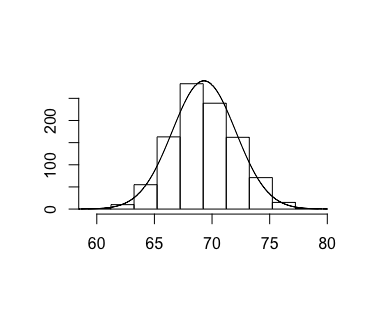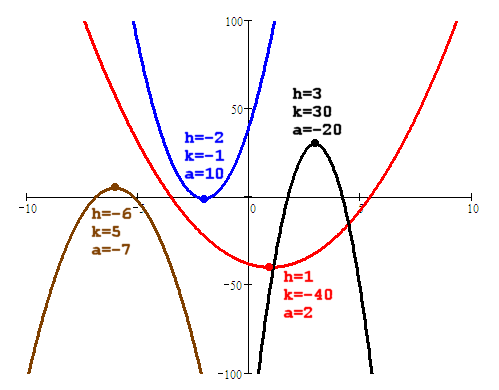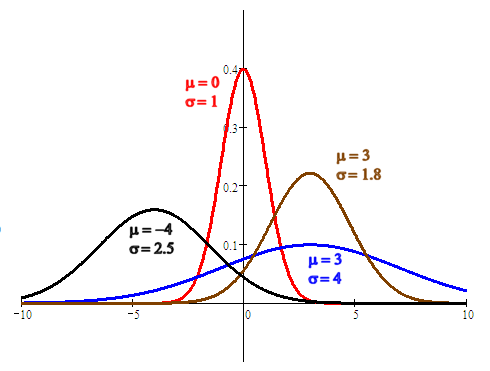## Parametric vs. Non-parametric Tests

Parametric tests deal with what you can say about a variable when you know (or assume that you know) its distribution belongs to a "known parametrized family of probability distributions".

Consider for example, the heights in inches of 1000 randomly sampled men, which generally follows a normal distribution with mean 69.3 inches and standard deviation of 2.756 inches.Note that knowing the values of the mean and standard deviation, tells us pretty much everything we need to know about this normal distribution. Indeed, recall the curve drawn above is a plot of the following function, whose values are completely determined by knowledge of $\mu$ and $\sigma$.

$$f(x) = \frac{e^{-\frac{1}{2} \left( \frac{x-\mu}{\sigma} \right)^2}}{\sigma \sqrt{2\pi}}$$

This is not disimilar to how the position and shape of graphs of quadratic functions of the following form depend only on the parameters of $a$, $h$, and $k$.

$$f(x) = a(x-h)^2+k$$

That is to say, knowing the values of these three parameters for any given quadratic function completely specifies the quadratic, telling us everything about the function (and its graph) that we wish to know (i.e., how to evaluate the function, where the vertex is, what is the direction of opening, what is the vertical stretching factor, etc...).Again, the same principle is at work with normal distributions. We can completely specify what a given Normal curve looks like by knowing just two parameters: $\mu$ (mu) and $\sigma$ (sigma).When we assume that the distribution of some variable (like heights of men in inches) follows a well-known distribution (like a normal distribution), that can be boiled down to knowledge of just a couple of parameters (like mu and sigma), and then we use that in conducting a hypothesis test, we are using a parametric test.

When you don't need to make such an assumption about the underlying distribution of a variable, to conduct a hypothesis test, you are using a nonparametric test. Such tests are more robust in a sense, but also frequently less powerful.

Because nonparametric tests don't require the typical assumptions about the nature of the underlying distributions that their parametric counterparts do, they are called "distribution free".

There are advantages and disadvantages to using non-parametric tests. In addition to being distribution-free, they can often be used for nominal or ordinal data. That said, they are generally less sensitive and less efficient too.

Frequently, performing these nonparametric tests requires special ranking and counting techniques.